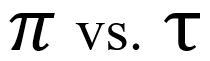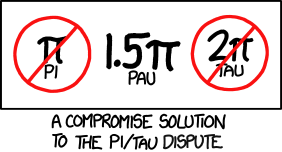#### Should we teach Tau instead of Pi?

by mbabbitt on Jun 16, 2021In the last 20 years, a growing movement has been calling for Pi (π or 3.14…) to be replaced by Tau (τ), which equals 2π or about 6.28. Students should be taught Tau, the argument goes, because it makes many concepts and equations more elegant and informative. And, the argument continues, nothing is lost by converting from Pi to Tau. Tau, like Pi, is defined by a relationship of measurements of a circle. Tau is the number of times you could place a string the length of a circle’s radius around the outside of that circle (see first Resources link below). Pi, of course, is the number of times you could do so with a string the length of the diameter. More traditionally, Pi is defined as the ratio of the circumference to the diameter (what you get when you divide the circumference by the diameter). Tau, instead, is the ratio of the circumference to the radius. So essentially the question is: is the radius or the diameter of a circle more important?

The leading proponents of Tau, like Michael Hartl, a physics professor at Caltech, and Bob Palais, a mathematics professor at the University of Utah, tend to be people who know high level math. Therefore the equations they champion as being more simple and elegant with Tau rather than Pi are often equations and formulas from high level physics and math. These include Cauchy’s integral formula, Fourier series formulas, Stirling’s approximation, Gaussian Normal Distribution, the Gauss-Bonnet and Picard theorems, Maxwell’s Equations (Gauss’s Law, Ampere’s Law, Coulomb’s Constant), and the reduced Planck’s constant (also known as Dirac’s Constant), and more. This list is taken from Palais’ 2001 article “π is wrong!”. Don’t worry, I also hardly know what any of that means. (Maybe your science teacher knows). However, I can explain why Tau enthusiasts list these formulas as arguments in their favor. Let’s take a simple one, Dirac’s constant. Dirac’s constant is h/2π (h is Planck’s constant, hence the alternate name “reduced Planck’s constant). If you replace 2π with Tau, you have h/τ. The equation looks simpler and less cluttered. You write less, but that’s not all. It also suggests that Tau is more essential to this concept. After all, Pi is only involved if you double it first. Imagine if you said 4 was more important than 8, but then you looked at a whole bunch of math and physics formulas and 8 kept showing up instead. You’d probably change your mind, right? So, by the same argument, Tau would seem to be more integral to numerous concepts found in mathematics and physics.

Those who argue for keeping Pi would point out that there is one major formula that has Pi without a 2: the area formula for a circle. A = πr^2. If we were to convert to Tau, the formula becomes A = ½τr^2. Why would you want to ruin such a basic and essential formula by introducing a fraction? I think this objection alone is enough for me to be unwilling to discard Pi, although I would not mind if it was taught alongside Tau. The area formula is taught at the middle school level and some circle questions can be quite difficult enough without having a fraction in the problem. Fans of Tau have a response to this objection. They say that the one half is similar to physics formulas (for example, free fall, kinetic energy, and potential energy of a spring) and that it reveals a connection to integration (a technique learned in AP Calculus). I see the merit of these arguments, but still would not want to subject 7th graders to an unnecessary fraction.

On the other hand, there is a concept that gives 11th and 12th graders headaches that would be radically transformed for the better if we adopted Tau. That concept is the unit circle. Hartl himself explains in this video why Tau is the superior choice for explaining the unit circle (it will start at about the 5 minute mark and you only need to watch until about the 7 minute mark). To quickly paraphrase, when you use 2π to represent a full turn of the circle, it is difficult to memorize angle measures. However, if you use Tau to represent one full turn, you now name an angle ⅛ of the way around the circle ⅛ Tau, an angle ¼ of the way as ¼ Tau, halfway around the circle is ½ Tau, etc.

Yes, switching to Tau makes sense, but, if I were still teaching precalculus, I probably would teach it alongside Pi. Tau is absent from most textbooks. It is not used on the AP exam. Calculators don’t have a Tau button, but they do have a Pi button. (Although the Google Calculator can evaluate expressions with Tau)!

The problem with switching to Tau is that Pi has the weight of tradition behind it. There would be a lot of work involved and, perhaps, not quite enough payoff. Also, Pi has Pi Day. You get to eat pie and celebrate math. Where as Tau Day (6/28) wouldn’t be during the school year.

I think math educators are a long way from adopting Tau and even further from throwing away Pi. I think that's good, but I also think students could benefit if we introduce Tau to older students and integrate the use of Tau into high school courses like precalculus and calculus and into college courses in math, engineering, physics, etc.

Or, we could follow the advice of Randall Munroe, author of the web-comic XKCD.One last thing, for the hardy few math-loving individuals who have read to this point: what about Euler’s identity? I know! I think e^iπ = -1 is the most beautiful formula in all of mathematics! However, it is not ruined by Tau. Instead, you would have the barely different e^iτ = 1. So rest easy. The universe’s most elegant equation is safe.

Resources:

A catchy song in favor of using Tau: YouTube: Vihart's A Song About A Circle Constant

The Tau Manifesto: https://tauday.com/tau-manifesto

Bob Palais’ article that started it all "Pi is wrong!"

http://taufortrig.org/ - resources for educators trying to use Tau to teach trig

Another video about Tau’s superiority with regards to the unit circle https://youtu.be/83ofi_L6eAo